# 读书笔记之：程序员面试宝典-1

1. 二进制位变换

x&(x-1)的结果是x最右边的1被置为0

2. 类型转换

float x=2.5f;

printf("%#x\n",(int&)x);//0x40200000(这个需要在C++下编译）
(int&)x效果等价于*(int*)&x,这是C++中可以编译通过（VC，g++都可以），在C中是不可以的。

#include <stdio.h>
int main(){
unsigned int a=0x77777777;
unsigned char i=(unsigned char)a;
char *b=(char*)&a;
char c=*(char*)&a;
printf("%#08x,%#08x,%#08x",i,*b,c);
return 0;
}

3.运算符符问题

#include <cstdio>
using namespace std;
int main(){
unsigned char a=0xA5;
unsigned char b=~a>>4+1;
printf("b=%d\n",b);
return 0;
}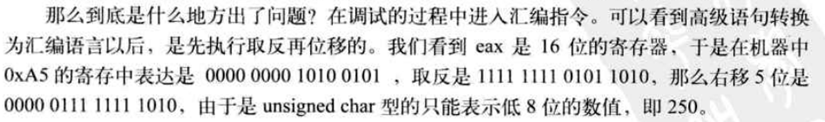4. x&(x-1)又一个应用实例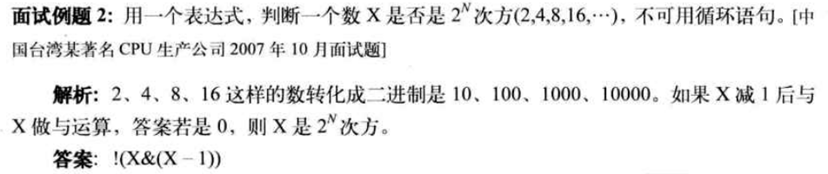1. 当两个数所有为 1 和为 0 的位都相同时，这两个数的平均值就是 (x & y) 。比如当 x 和 y 都等于 1100 时，x & y 的值也是 1100 ，我们也可以说此时求 x 和 y 的平均数可以用 (x & y) 来求得。
2. 当两个数中的所有对应位有且只有一个为 1 时，那么这两个数的平均值由 (x^y)>>1 表达式求得。比如当 x 为 101100 (十进制 44)，y 为 010010 (十进制 18) 时，x ^ y 的值为 111110 ，然后再将 111110 向右移动 1 位后得 11111 (十进制为 31)，也就是 (44 + 18)/2 = 31 。

x 的二进制数位 1100 ，y 的二进制数为 11000 。我们先将 x 和 y 做相与运算：
01100
11000        &
--------------
01000

01100
11000  ^
-----------
10100

5.  比较两个数

int max=(a+b+abs(a-b))/2;

int flag=(((a - b) >> (8 * sizeof(int)  - 1))) & 0x01; //获得两者差的符号位
int buf={a,b};
int max=buf[flag];//如果flag=0，说明a>b，否则a<b

int max=(flag * b) + ((1 - flag) * a);

int test1(){
int x,y;
int z;
int i;
double m;
srand((int)time(NULL));
for(i=0;i<10;i++)
{
x=rand()%100;
y=rand()%100;
m=(x+y)/2.0;
z=(x&y)+((x^y)>>1);
printf("%4d%4d%5.1f%4d%4d%4d\n",x,y,m,x&y,x^y,z);
}
}
int test2(){
int i;
srand((int)time(NULL));
for(i=0;i<10;i++){
int x=rand()%100;
int y=rand()%100;
int buf={x,y};
unsigned int z;
z=x-y;
z>>=31;
printf("%3d%3d%3d\n",x,y,buf[z]);
}
return 0;
}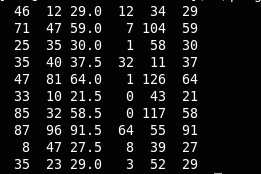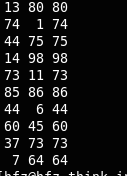6. 删除C/C++程序中的注释

7. const用法，在C与C++中的不同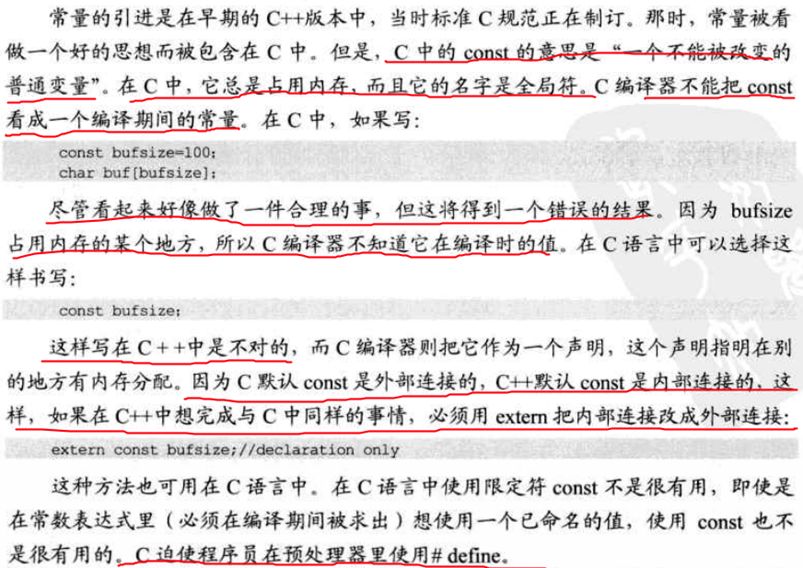8. 内存对齐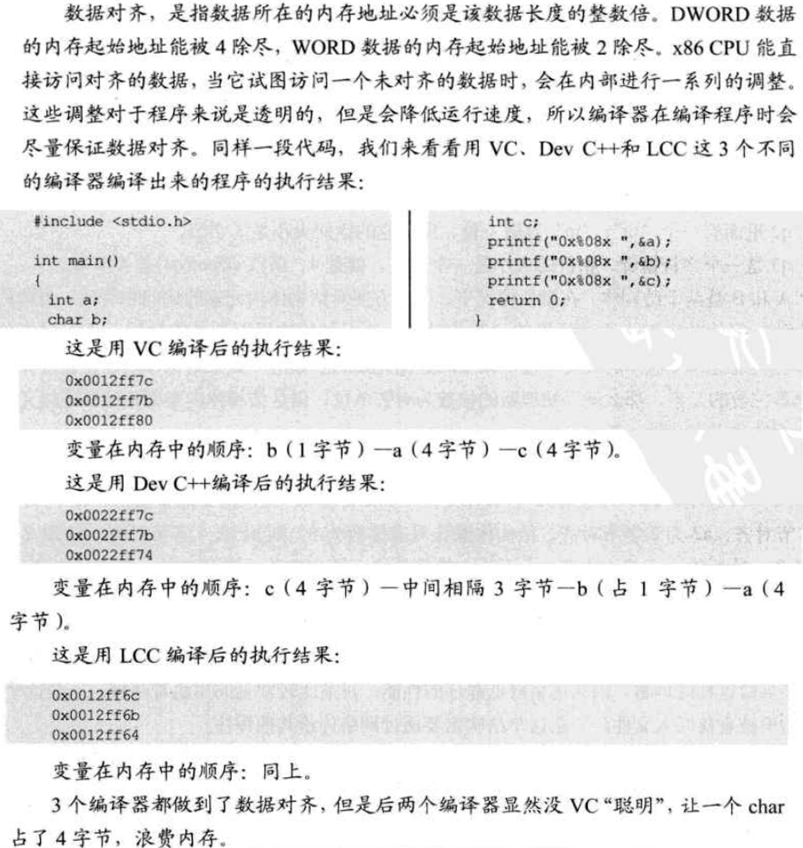int main(){
int a;
char b;
int c;
char *p=(char*)&a;
printf("%#08x\n",&a);
printf("%#08x\n",&b);
printf("%#08x\n",&c);
printf("%#08x\n",p);
printf("%#08x\n",p+1);
printf("%#08x\n",p+2);
printf("%#08x\n",p+3);

return 0;
}

gcc编译运行如下：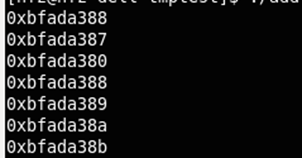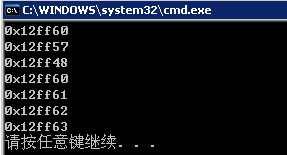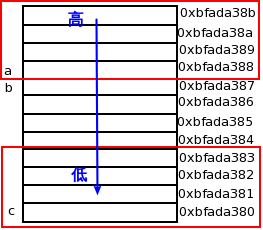http://www.cnblogs.com/xkfz007/archive/2012/06/22/2558935.html

int test3(){

int i=-1,a[]={1,2,3,4,5};
char *p=NULL;
printf("%#08x\n",&i);
printf("%#08x\n",a);
printf("%#08x\n",&p);
for(i=0;i<5;i++){
printf("%d: ",i);
p=(char*)&a[i];
printf("%#08x ",p);
printf("%#08x ",p+1);
printf("%#08x ",p+2);
printf("%#08x\n",p+3);

}
}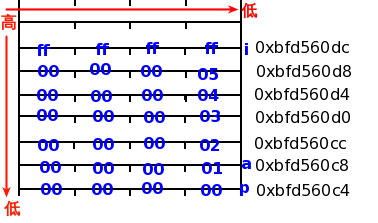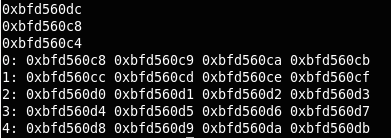9. C++中类也遵循内存对齐

10. sizeof空类，多重继承

11. 指针减法运算

#include <stdio.h>
#include <stdlib.h>
int main(int argc, char *argv[])
{
int arr = {1,2,3,4,5};
int *ptr = arr;
printf("%d\n",ptr-ptr);

return 0;
}

《C 和指针》P110 分析如下：两个指针相减的结果的类型为ptrdiff_t,它是一种有符号整数类型。减法运算的值为两个指针在内存中的距离（以数组元素的长度为单位，而 非字节），因为减法运算的结果将除以数组元素类型的长度。所以该结果与数组中存储的元素的类型无关。

size_t是unsigned类型，用于指明数组长度或下标，它必须是一个正数，std::size_t
ptrdiff_t是signed类型，用于存放同一数组中两个指针之间的差距，它可以使负数，std::ptrdiff_t.
size_type是unsigned类型,表示容器中元素长度或者下标，vector<int>::size_type i = 0;
difference_type是signed类型,表示迭代器差距，vector<int>:: difference_type = iter1-iter2.

12. 获取成员变量偏移量的两种方式
第一种方式是MFC里使用广泛的宏：#define OFFSET(structure, member) ((int)&((structure*)0)->member); 正如我们平时通过某对象的地址指针访问某个成员变量一样，这里只是强制使用0作为该地址，但区别是并没有通过该地址去访问成员变量，而只是用&操 作符来获取该成员变量的地址，所以不会出现访问违规的情况。所以，完全可以用此类声明一个对象，然后用该对象某成员变量地址减去该对象首地址获取偏移量， 只是纯虚类无法这样实现。
另一种方式是通过域操作符取成员变量的地址。例如一个类Test有int 型成员变量x，则可以通过int Test::* pOffset = &Test::x 获得该偏移量，然后通过int nOffset = reinterpret_cast<int>(*(void**)(&pOffset))将其转化为整型量。
另外，以上这两种方式都对静态成员无效。

class A{
public:
A():m_a(1),m_b(2){
cout<<"A()"<<endl;
}
~A(){
cout<<"~A()"<<endl;
}
void fun(){
cout<<"A:fun "<<m_a<<" "<<m_b<<" "<<endl;
}
public:
int m_a;
int m_b;
static int s_a;
};
int A::s_a=3;
class B{
public:
B():m_c(3){
cout<<"B()"<<endl;
}
~B(){
cout<<"~B()"<<endl;
}
void fun(){
cout<<"B:fun "<<m_c<<endl;
}
public:
int m_c;
};
void test1(){
A a;
B *p=(B*)(&a);
cout<<"&a:"<<&a<<endl;
cout<<"&(a.m_a):"<<&(a.m_a)<<endl;
cout<<"&(a::s_a)"<<&a.s_a<<endl;
cout<<"&(A::s_a)"<<&A::s_a<<endl;
int A::* q=&A::m_a;
cout<<"&A::m_a:"<<reinterpret_cast<int>(*(void**)(&q))<<endl;
q=&A::m_b;
cout<<"&A::m_b:"<<reinterpret_cast<int>(*(void**)(&q))<<endl;
int B::* t=&B::m_c;
cout<<"&B::m_c:"<<reinterpret_cast<int>(*(void**)(&t))<<endl;
p->fun();
}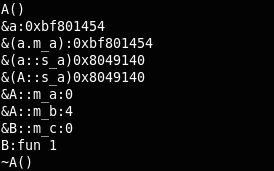13. 指针与句柄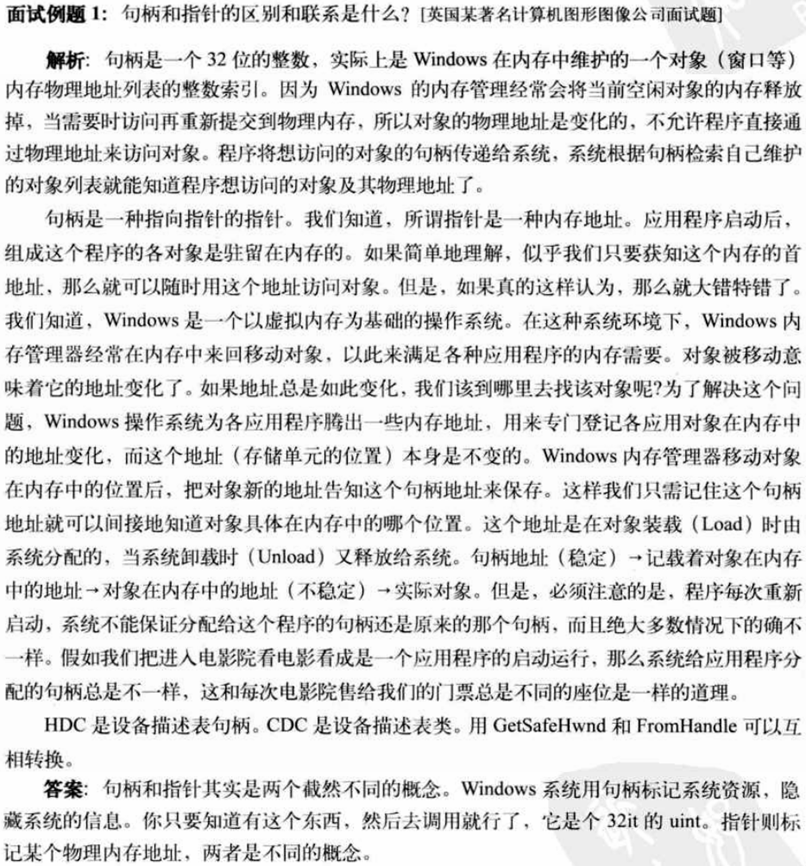14. 输入两个字符串，比如abdbcc和abc，输出第二个字符串在第一个字符串中的连接次序，即输出125，146，145，146.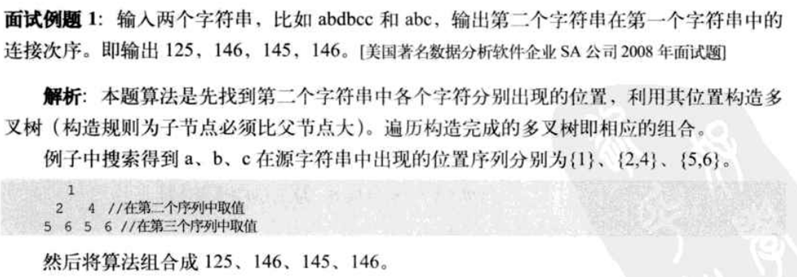View Code
#include <iostream>
#include <cstdio>
#include <cstring>
using namespace std;
void PrintArray(char *p_str,char*s_str,
int *print_arr,int p_len,
int s_len,int print_arr_num,
int p_start_num,int s_start_num){
int pStartnum=p_start_num,
sStartnum=s_start_num;
int printNum=print_arr_num;
if(printNum==s_len){
for(int i=0;i<s_len;i++)
cout<<*(print_arr+i)<<' ';
cout<<endl;
return;
}
for(int i=pStartnum;i<p_len;i++){
for(int j=sStartnum;j<s_len;j++){
if(*(p_str+i)==*(s_str+j)) {
print_arr[printNum]=i+1;
pStartnum=i;
sStartnum=j;
PrintArray(p_str,s_str,
print_arr,p_len,s_len,
printNum+1,pStartnum+1,
sStartnum+1);
}
}
}
}
void ConnectSequence(char* p_str,char *s_str){
if(NULL==p_str&&NULL==s_str){
cout<<"string error"<<endl;
return ;
}
int p_len=strlen(p_str);
int s_len=strlen(s_str);
int *print_arr=new int[s_len];
unsigned int print_arr_num=0;
if(NULL==print_arr){
cout<<"allocate error"<<endl;
return ;
}
PrintArray(p_str,s_str,print_arr,p_len,s_len,0,0,0);
}
int main(){
char parStr[]="abdbcca";
char sonStr[]="abc";
ConnectSequence(parStr,sonStr);
return 0;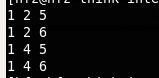15. 给出如下递归表达式的非递归计算方法：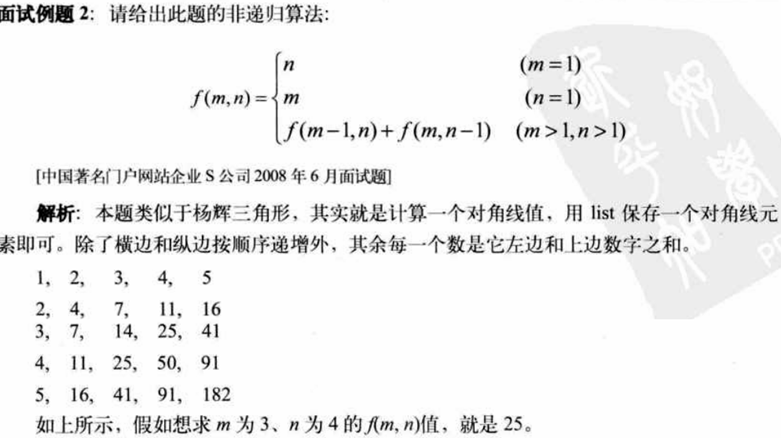View Code
#include <iostream>
#include <cstdlib>
using namespace std;
long long f(int m,int n){
if(1==m)
return n;
else if(1==n)
return m;
else
return f(m-1,n)+f(m,n-1);
}
long long f2(int m,int n){
const int M=m;
const int N=n;
long long a[M][N];
for(int i=0;i<M;i++)
a[i]=i+1;
for(int j=1;j<N;j++)
a[j]=j+1;
for(int i=1;i<M;i++)
for(int j=1;j<N;j++)
a[i][j]=a[i-1][j]+a[i][j-1];
return a[M-1][N-1];
}
int main(){
for(int i=0;i<10;i++){
int m=rand()%8;
int n=rand()%8;
long long y=f(m,n);
long long y2=f2(m,n);
cout<<'<'<<m<<','<<n<<""<<y<<' '<<y2<<endl;
}
}

16. zig-zag扫描问题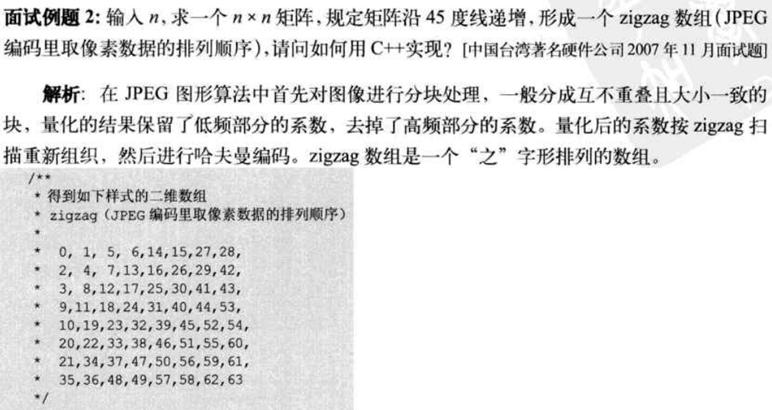实现代码如下：View Code
#include <iostream>
#include <iomanip>
using namespace std;
void zigzag(int n){
const int N=n;
int a[N][N];
int squa=N*N;
int b[N*N];
for(int i=0;i<N;i++)
for(int j=0;j<N;j++){
int s=i+j;
if(s<N) {
a[i][j]=s*(s+1)/2+((i+j)%2==0?j:i);
}
else{
s=(N-1-i)+(N-1-j);
a[i][j]=squa-s*(s+1)/2-(N-((i+j)%2==0?j:i));
}
b[a[i][j]]=i;
b[a[i][j]]=j;
}
for(int i=0;i<N;i++){
for(int j=0;j<N;j++)
cout<<setw(2)<<a[i][j]<<" ";
cout<<endl;
}
for(int i=0;i<N*N;i++) {
for(int j=0;j<2;j++)
cout<<setw(2)<<b[i][j]<<' ';
cout<<endl;
}
}
int main(){
int n=4;
zigzag(n);
}

17. 两个数组匹配的问题：

A，B两个数组是等长度，但顺序不同，对两个数组中的数进行匹配。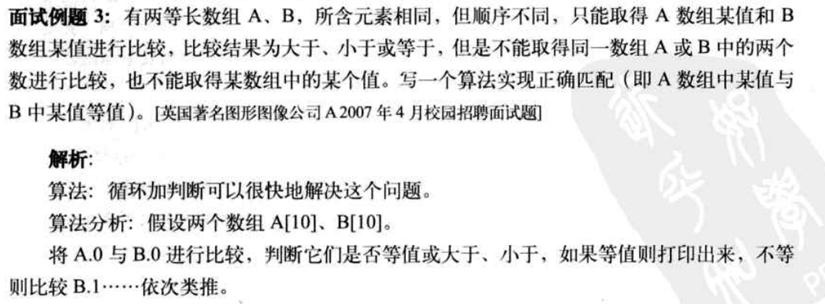View Code
#include <iostream>
using namespace std;
void match(int a[],int b[],int k){
for(int i=0;i<k;i++){
for(int j=0;j<k;j++){
if(a[i]==b[j]){
cout<<"a["<<i<<"] match b["<<j<<"]"<<endl;
break;
}
}
}
}
int main(){
int a={1,2,3,4,5,6,7,8,9,10};
int b={10,6,4,5,1,8,7,9,3,2};
int k=sizeof(a)/sizeof(int);
match(a,b,k);
return 0;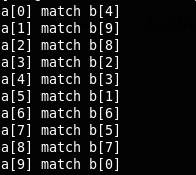18. 螺旋队列问题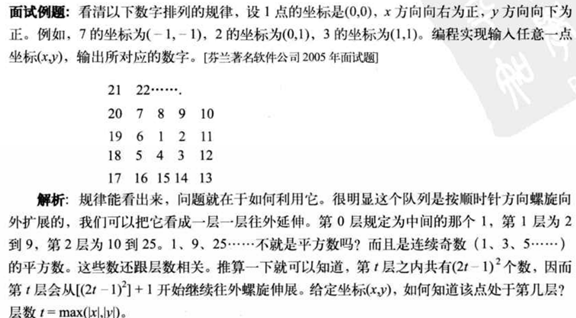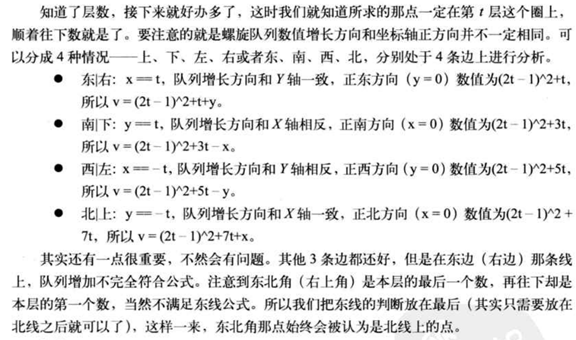View Code
#include <iostream>
#include <iomanip>
#define max(a,b) ((a)>(b)?(a):(b))
#define abs(a) ((a)>0?(a):-(a))
using namespace std;
int spiral(int x,int y){
int t=max(abs(x),abs(y));
int u=t+t;
int v=u-1;
v=v*v+u;
if(x==-t)
v+=u+t-y;
else if(y==-t)
v+=3*u+x-t;
else if(y==t)
v+=t-x;
else
v+=y-t;
return v;
}
int main(){
for(int i=-4;i<=4;i++){
for(int j=-4;j<=4;j++)
cout<<setw(3)<<spiral(j,i)<<' ';
cout<<endl;
}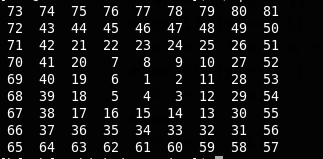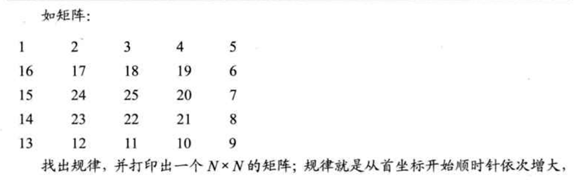View Code
#include <iostream>
#include <iomanip>
#define max(a,b) ((a)>(b)?(a):(b))
#define abs(a) ((a)>0?(a):-(a))
using namespace std;
int spiral(int x,int y){
int t=max(abs(x),abs(y));
int u=t+t;
int v=u-1;
v=v*v+u;
if(x==-t)
v+=u+t-y;
else if(y==-t)
v+=3*u+x-t;
else if(y==t)
v+=t-x;
else
v+=y-t;
return v;
}
void test1(){
for(int i=-4;i<=4;i++){
for(int j=-4;j<=4;j++)
cout<<setw(3)<<spiral(j,i)<<' ';
cout<<endl;
}

}
int a;
void spiral2(int n){
int m=1;
for(int i=0;i<n/2;i++){
for(int j=0;j<n-i;j++){
if(a[i][j]==0)
a[i][j]=m++;
}
for(int j=i+1;j<n-i;j++){
if(a[j][n-1-i]==0)
a[j][n-1-i]=m++;
}
for(int j=n-i-1;j>i;j--){
if(a[n-i-1][j]==0)
a[n-1-i][j]=m++;
}
for(int j=n-i-1;j>i;j--) {
if(a[j][i]==0)
a[j][i]=m++;
}
}
if(n%2)
a[n/2][n/2]=m;
}
int test2(){
const int n=10;
for(int i=0;i<n;i++)
for(int j=0;j<n;j++)
a[i][j]=0;
spiral2(n);
for(int i=0;i<n;i++){
for(int j=0;j<n;j++)
cout<<setw(3)<<a[i][j]<<' ';
cout<<endl;
}
}
int main(){
test2();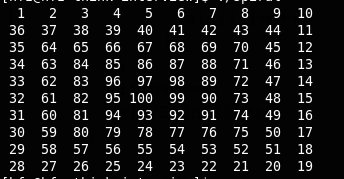19. 概率问题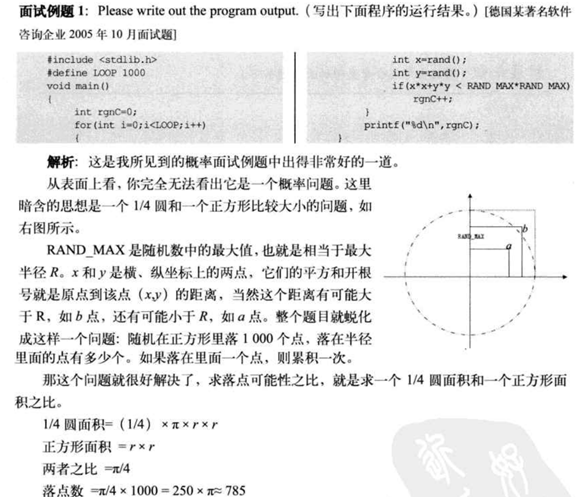View Code
#include <iostream>
#include <cstdlib>
using namespace std;
int main(){
const int Loop=1000;
const int R=100;
for(int i=0;i<=500;i++){
int rgn=0;
for(int j=0;j<Loop;j++){
int x=rand()%R;
int y=rand()%R;
if(x*x+y*y<R*R)
rgn++;
}
cout<<rgn<<' ';
if((i+1)%20==0)
cout<<endl;
}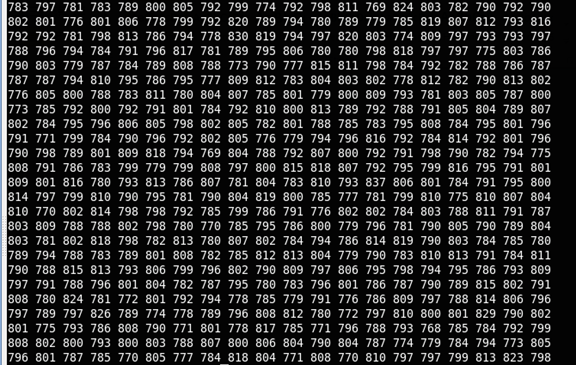20. 虚函数，覆盖问题View Code
#include <iostream>
using namespace std;
class A{
protected:
int m_data;
public:
A(int data=0):m_data(data){}
int GetData(){
cout<<"A-1 ";
return doGetData();
}
int virtual doGetData(){
//        int doGetData(){
cout<<"A-2 ";
return m_data;
}
};
class B:public A{
protected:
int m_data;
public:
B(int data=1):m_data(data){}
int doGetData(){
cout<<"B-2 ";
return m_data;
}
};
class C:public B{
protected:
int m_data;
public:
C(int data=2){
m_data=data;
}
};
int main1(){
C c(10);
cout<<c.GetData()<<endl;
cout<<c.A::GetData()<<endl;
cout<<c.B::GetData()<<endl;
cout<<c.C::GetData()<<endl;
cout<<c.doGetData()<<endl;
cout<<c.A::doGetData()<<endl;
cout<<c.B::doGetData()<<endl;
cout<<c.C::doGetData()<<endl;
return 0;

}
class AA{
public:
virtual void f(){
cout<<"AA "<<endl;
}

};
class BB:public AA{
public:
void f(){
cout<<"BB "<<endl;
}
};
int main(){
AA* pa=new AA();
pa->f();
BB* pb=(BB*)pa;
pb->f();
delete pa,pb;
pa=new BB();
pa->f();
pb=(BB*)pa;
pb->f();
return 0;

21. 虚函数继承与虚继承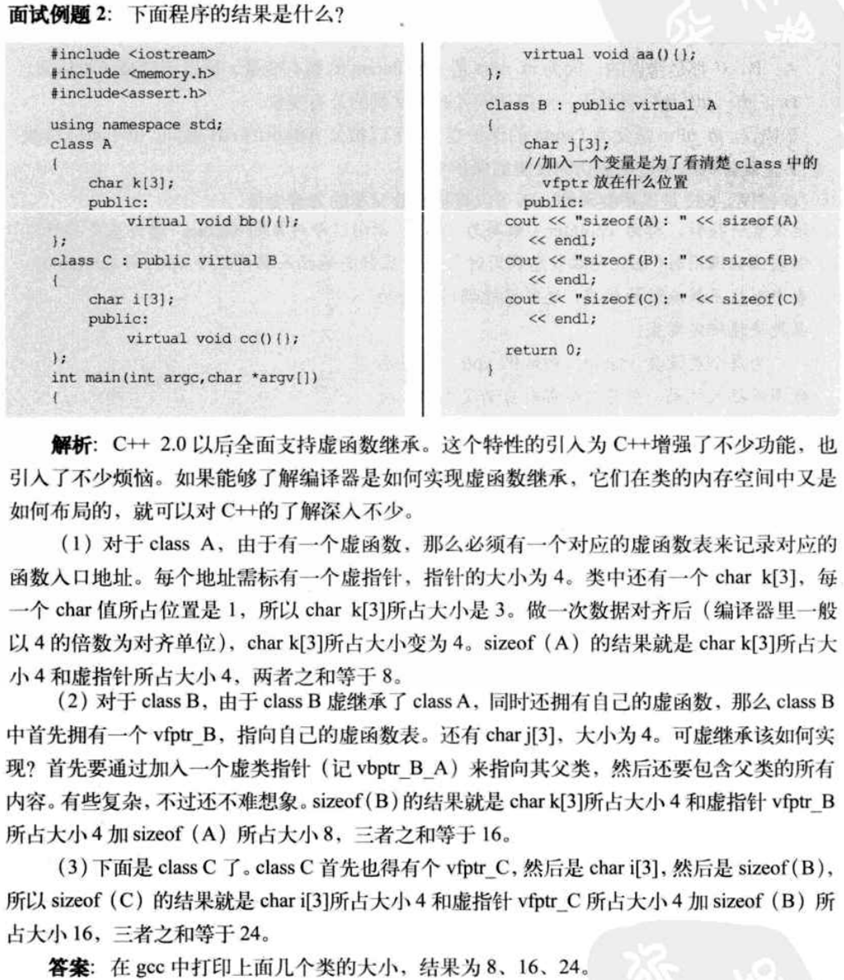22. 多重继承 的优点与缺陷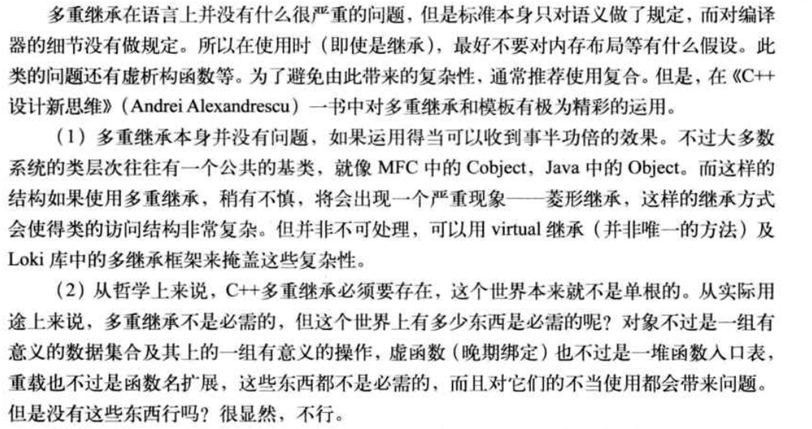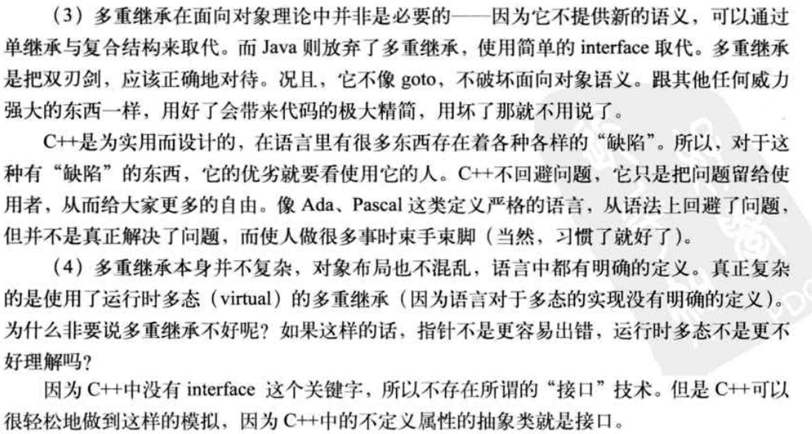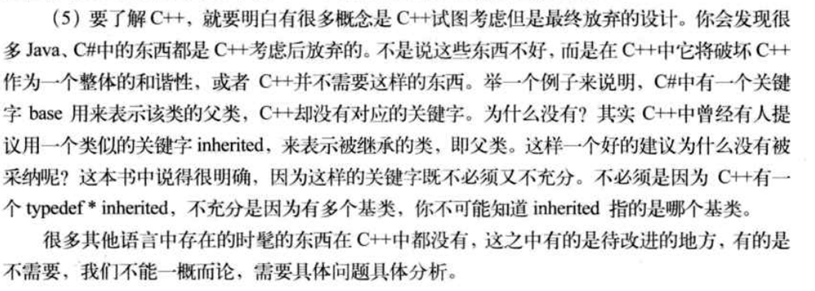23. C++所引入的额外开销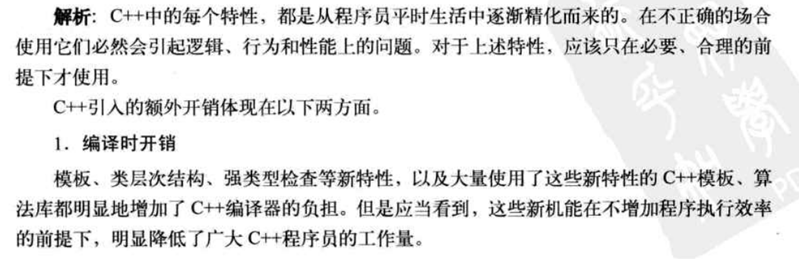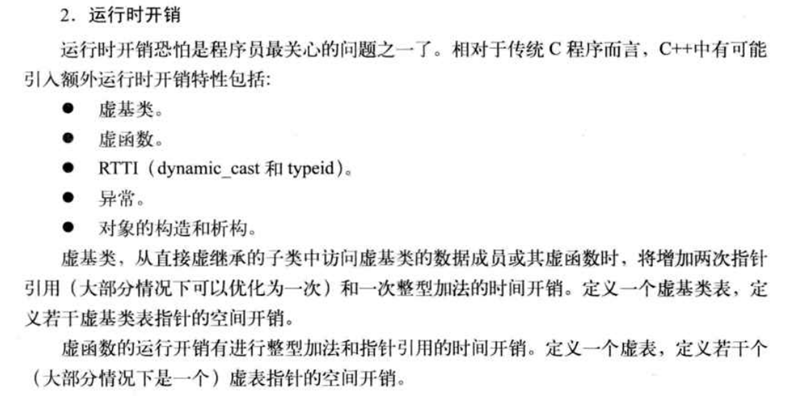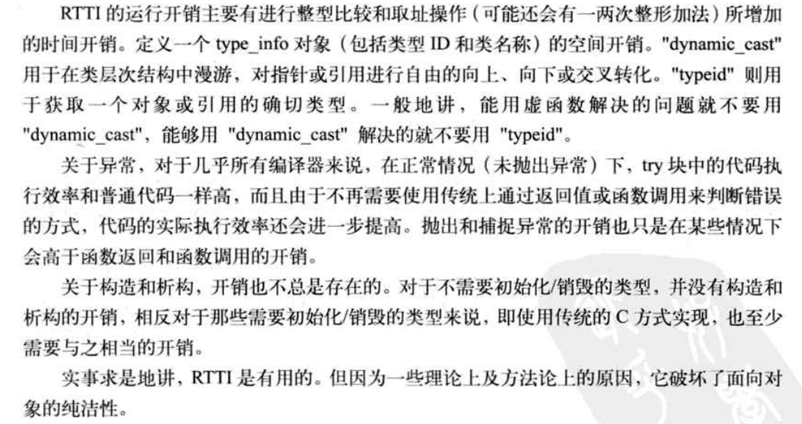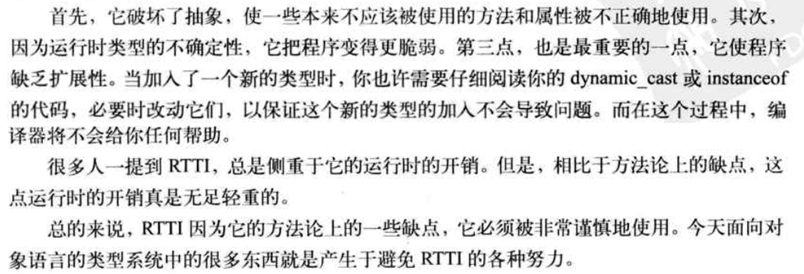posted @ 2012-07-16 13:14  Mr.Rico  阅读(...)  评论(...编辑  收藏